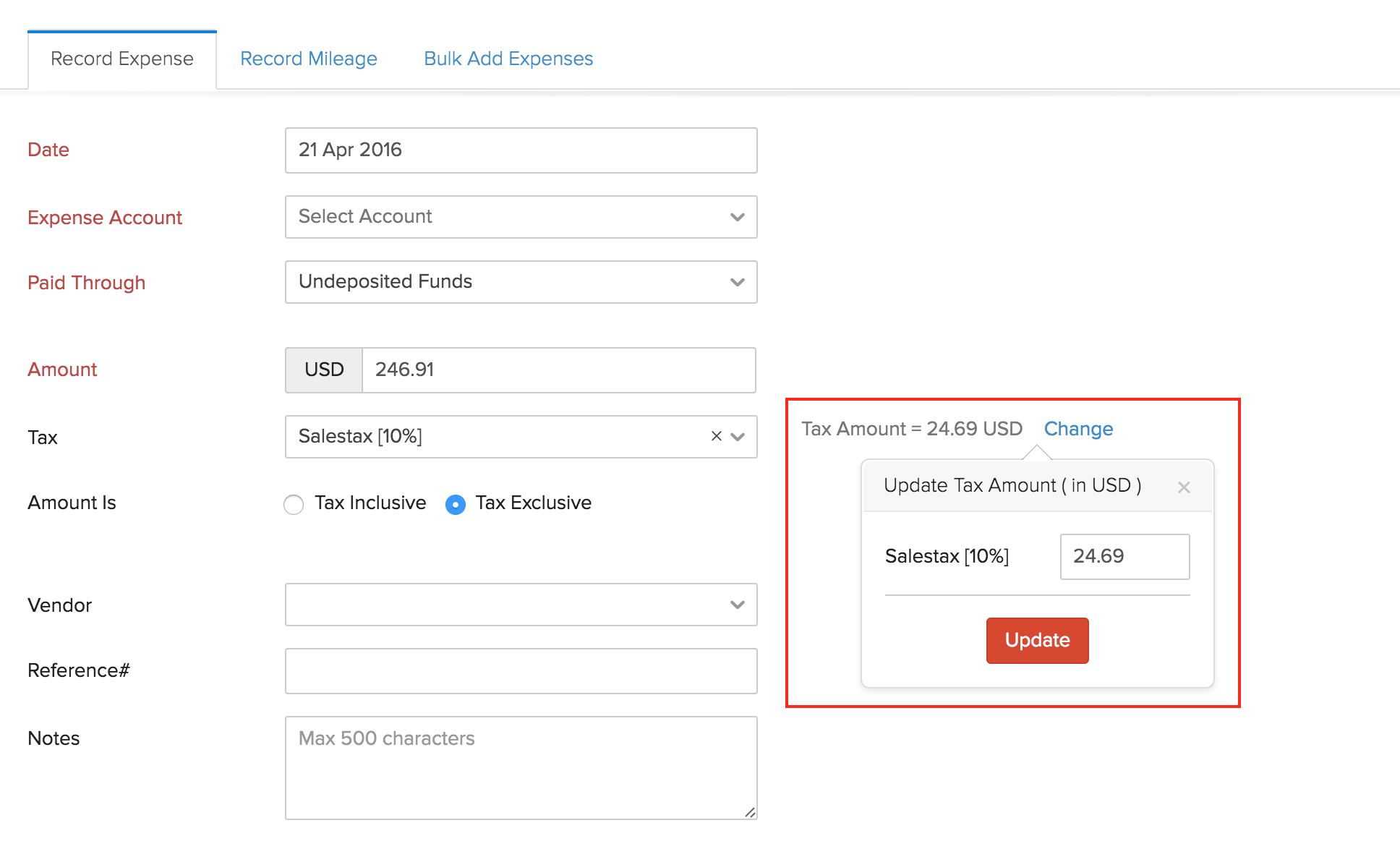FAQ

How do I manually change the tax amount for an expense?

There might be cases wherein the tax amount in a transaction, say an expense, varies because your vendor calculates the tax applied on the transaction differently.

Zoho Invoice allows you to account for these minor variations by allowing you to manually change i.e override an automatically calculated tax amount, once you apply a tax to a transaction.

Let’s take an example where you print two sets of flyers, A5 (100 Nos.) and A4 (50 Nos.) to give it out to customers at a Sales conference.

The receipt of the expense has the following details:

A5 Flyer (100 Nos.) - \$ 123.45

A4 Flyer (50 Nos. ) - \$ 123.46

Sub Total - \$ 246.91

The vendor also adds a 10% tax for every item in the bill.

10% of 123.45 = 12.345 => 12.35 (Rounded for 2 decimal places)

10% of 123.46 = 12.346 => 12.35 (Rounded for 2 decimal places)

Now tax amount will be 12.35+12.35 = \$24.70

Total - \$ 246.91 + \$ 24.70 = \$ 271.61

However, when you attach the receipt and add the expense details in Zoho Invoice, you will enter the sub total of \$ 246.91 and you will choose the tax of 10% from the drop-down (or create a new one) and mark it as Tax Exclusive.

Now, the tax in Zoho Invoice will be calculated for the whole amount (\$ 246.91) rather than how it was done by the vendor (apply tax to each item). This would result in a tax amount of \$24.69.

From the above calculation you can observe a small variation between the calculations done by the vendor and Zoho Invoice.

Under such cases, you can maually adjust these variations using the Tax override feature.

In this case, all you have to do is manually change the tax amount that was initially calculated to reflect the actual tax amount spent.

While creating an expense, click on the Change option next to the Tax Amount to modify the tax rate that has been calculated automatically.Finally, click on the Save button to save the transaction with the modified tax rate.

P.S.: This option is currently available only for the Expenses module.YesNoThank you for your feedback!